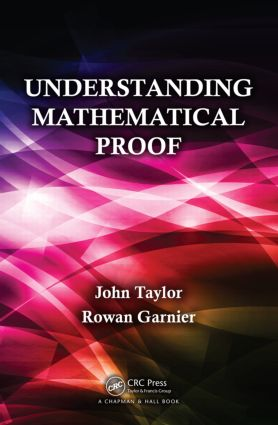# Understanding Mathematical Proof

## 1st Edition

Chapman and Hall/CRC

414 pages | 62 B/W Illus.

##### Purchasing Options:\$ = USD
Paperback: 9781466514904
pub: 2014-03-21
SAVE ~\$14.19
\$70.95
\$56.76
x
Hardback: 9781138466852
pub: 2018-06-28
SAVE ~\$39.00
\$195.00
\$156.00
x
eBook (VitalSource) : 9780429190735
pub: 2016-04-19
from \$34.48

FREE Standard Shipping!

### Description

The notion of proof is central to mathematics yet it is one of the most difficult aspects of the subject to teach and master. In particular, undergraduate mathematics students often experience difficulties in understanding and constructing proofs.

Understanding Mathematical Proof describes the nature of mathematical proof, explores the various techniques that mathematicians adopt to prove their results, and offers advice and strategies for constructing proofs. It will improve students’ ability to understand proofs and construct correct proofs of their own.

The first chapter of the text introduces the kind of reasoning that mathematicians use when writing their proofs and gives some example proofs to set the scene. The book then describes basic logic to enable an understanding of the structure of both individual mathematical statements and whole mathematical proofs. It also explains the notions of sets and functions and dissects several proofs with a view to exposing some of the underlying features common to most mathematical proofs. The remainder of the book delves further into different types of proof, including direct proof, proof using contrapositive, proof by contradiction, and mathematical induction. The authors also discuss existence and uniqueness proofs and the role of counter examples.

### Reviews

"The book is written in a precise and clear style, with lots of appropriately chosen examples and a sufficient amount of (clear) diagrams. … could be useful to, and enjoyed by, students seeking a concise introduction to the notion of mathematical proof."

London Mathematical Society Newsletter, No. 454, January 2016

"The manner in which the authors expose their ideas is a very kind and easy to understand one. The book contains lots of examples and comments. Far more, all the judgements are well exposed. The examples that are offered cover a large area of elementary mathematics, such as calculus, logic, sets and functions, linear algebra, and group theory. We highly recommend this book, first of all to those who study mathematics, but we also find it useful for those who study engineering and computer science."

Zentralblatt MATH 1311

### Table of Contents

Introduction

The need for proof

The language of mathematics

Reasoning

Deductive reasoning and truth

Example proofs

Logic and Reasoning

Introduction

Propositions, connectives, and truth tables

Logical equivalence and logical implication

Predicates and quantification

Logical reasoning

Sets and Functions

Introduction

Sets and membership

Operations on sets

The Cartesian product

Functions and composite functions

Properties of functions

The Structure of Mathematical Proofs

Introduction

Some proofs dissected

An informal framework for proofs

Direct proof

A more formal framework

Finding Proofs

Direct proof route maps

Examples from sets and functions

Examples from algebra

Examples from analysis

Direct Proof: Variations

Introduction

Proof using the contrapositive

Proof of biconditional statements

Proof of conjunctions

Proof by contradiction

Further examples

Existence and Uniqueness

Introduction

Constructive existence proofs

Non-constructive existence proofs

Counter-examples

Uniqueness proofs

Mathematical Induction

Introduction

Proof by induction

Variations on proof by induction

Hints and Solutions to Selected Exercises

Bibliography

Index

### Subject Categories

##### BISAC Subject Codes/Headings:
MAT000000
MATHEMATICS / General
MAT028000
MATHEMATICS / Set Theory